##### Differential Equations For Dummies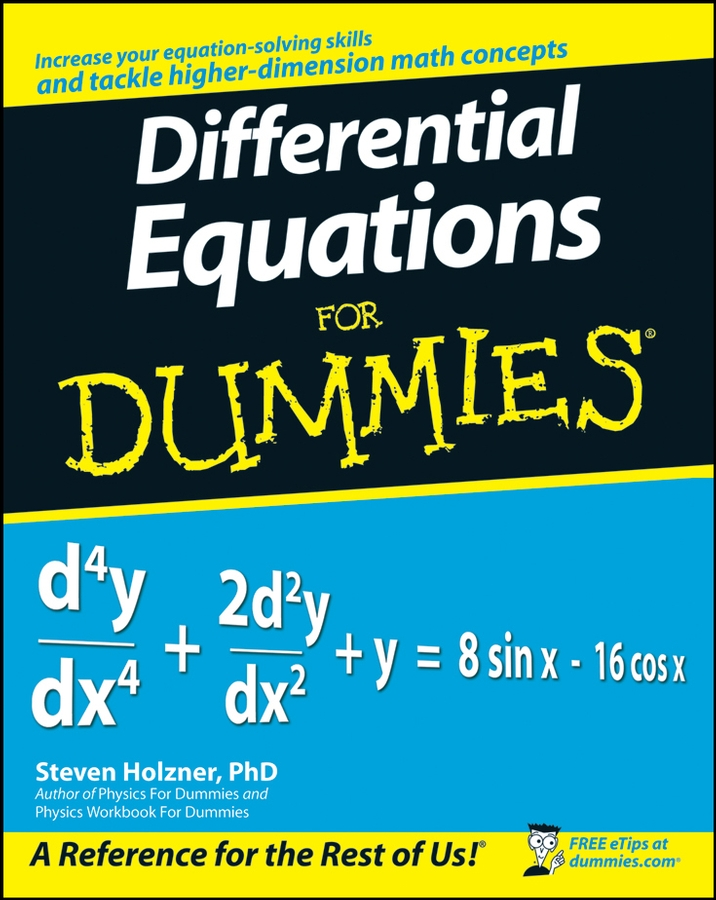Some differentiation rules are a snap to remember and use. These include the constant rule, power rule, constant multiple rule, sum rule, and difference rule.
• The constant rule: This is simple. f (x) = 5 is a horizontal line with a slope of zero, and thus its derivative is also zero.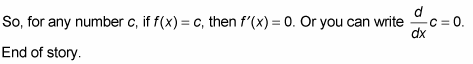• The power rule: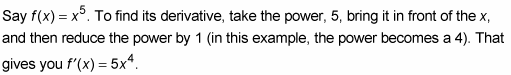To repeat, bring the power in front, then reduce the power by 1. That’s all there is to it.

The power rule works for any power: a positive, a negative, or a fraction.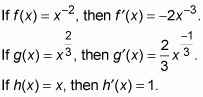Make sure you remember how to do the last function. It’s the simplest function, yet the easiest problem to miss. By the way, do you see how finding this last derivative follows the power rule? (Hint: x to the zero power equals one).

You can differentiate radical functions by rewriting them as power functions and then using the power rule.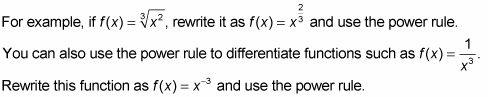• The constant multiple rule: What if the function you’re differentiating begins with a coefficient? Makes no difference. A coefficient has no effect on the process of differentiation. You just ignore it and differentiate according to the appropriate rule. The coefficient stays where it is until the final step when you simplify your answer by multiplying by the coefficient.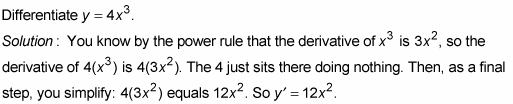Don’t forget that ð (~3.14) and e (~2.72) are numbers, not variables, so they behave like ordinary numbers. Constants in problems, like c and k, also behave like ordinary numbers. So, for example,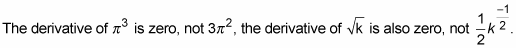• The sum rule: When you want the derivative of a sum of terms, take the derivative of each term separately.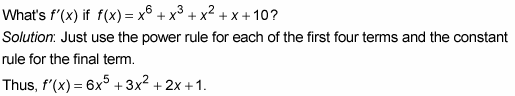• The difference rule: If you have a difference (that’s subtraction) instead of a sum, it makes no difference. You still differentiate each term separately.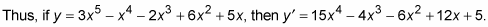The addition and subtraction signs are unaffected by the differentiation.